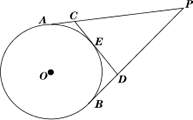Friday 05th June 2020CBSE Guess > Papers > Important Questions > Class X > 2014 > Mathematics > Math-index > Mathematics By Mr. Ajai Kumar Shukla CBSE CLASS X Mathematics MATHEMATICS Max Marks  40 Time  1 ½ hours. SECTION A (EACH Q. 1 MARKS) Find the roots of the equation 3x2 -5x + 2 = 0 Find the values of k for which the equation (k+1) x2 – 2xk+2x+1=0 has real & equal roots? If the sum of first m terms of an A.P. is n & the sum of its first n terms is m, then find the sum of its first (m + n) terms?  Write the formula for sum of first n terms of an A.P. whose first term is a & the last is l? Find the sum of first 15 terms of an A.P. whose nth term is 9 – 5n A point T is 13 cm away from the centre of a circle. The length of the tangent drawn from T to the circle is 12 cm. Find the radius of the circle. Find the length of the tangent drawn from a point P whose distance from the centre of the circle is 25cm. It is given that radius of the circle is 7cm. What is the distance between the points A(c, 0) & B(0,-c)?  Find x if the distance between the points (x ,2) & (3,4) be 8 units. Find the mid-point of the line segment joining the points (4, 3) & (-2,1). SECTION "B" (EACH Q. 3 MARKS) A vertical flagstaff stands on a horizontal plane. From a point 200m from its foot, the angle of elevation of its top is found to be 300.Find the height of the flagstaff. The upper part of a tree broken over by the wind makes an angle  of 300  with the ground and the  distance of the root from the point where the top touches the ground is 25 m. What was the height of the tree An observer 1.5 m tall is 28.5 m away from a tower 30 m high. Determine the angle of elevation  Prove that the angle between the two tangents drawn from an external point to a circle is supplementary to the angle subtended by the line segment joining the point of contact at the centre. If all the sides of a parallelogram touch a circle, then show that the parallelogram is a rhombus. The 8th term of an A.P is zero. Prove that its 38th term is triple of its 18th term. Which term of the A.P.5,9,13, is 81? Find also the sum of 5+9+13+--------+81. The sum of the squares of two numbers is 130. The sum of the smaller number and twice the larger number is 25. Find the numbers. The sum of age of a son & his father is 35 & the product of their ages is 150. Find their ages. Find two consecutive numbers whose squares have the sum 85. Which term of AP : 3, 8, 13, 18, ......, is 78? From an external point P, tangents PA and PB  are down to a circle with centre O. If CD is the tangent to the circle at a point E and PA=14cm, find the perimeter ofCBSE Important Questions Class X Mathematics By : Mr. Ajai Kumar Shukla Submitted By : Mr. Ajai Kumar Shukla, RACHNA TUTORIALS, 6/1003 JANKIPURAM VISTAR LUCKNOW Mobile : +91 - 9415467421 Email Id : [email protected]